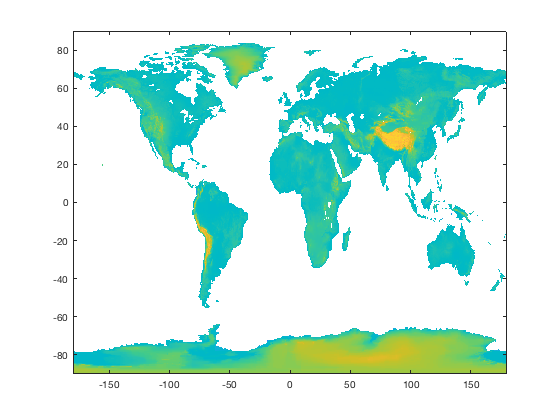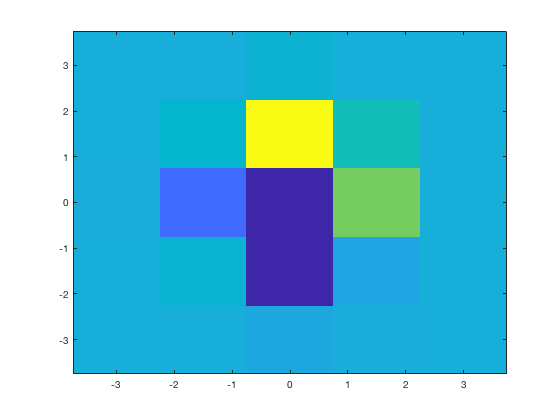# imagescn documentation

The imagescn behaves just like imagesc, but makes NaNs transparent, sets axis to xy if xdata and ydata are included, and has more error checking than imagesc.

Back to Climate Data Tools Contents

## Syntax

```imagescn(C)
imagescn(x,y,C)
imagescn(x,y,C,clims)
imagescn('PropertyName',PropertyValue,...)
h = imagescn(...)```

## Description

imagescn(C) displays the data in array C as an image that uses the full range of colors in the colormap. Each element of C specifies the color for 1 pixel of the image. The resulting image is an m-by-n grid of pixels where m is the number of columns and n is the number of rows in C. The row and column indices of the elements determine the centers of the corresponding pixels. NaN values in C appear transparent.

imagescn(x,y,C) specifies x and y locations of the centers of the pixels in C. If x and y are two-element vectors, the outer rows and columns of C are centered on the values in x and y. Mimicking imagesc, if x or y are vectors with more than two elements, only the first and last elements of of the vectors are considered, and spacing is automatically scaled as if you entered two-element arrays. The imagescn function takes this one step further, and allows you to enter x and y as 2D grids the same size as C. If x and y are included, the imagescn function automatically sets axes to cartesian xy rather than the (reverse) ij axes.

imagescn(x,y,C,clims) specifies the data values that map to the first and last elements of the colormap. Specify clims as a two-element vector of the form [cmin cmax], where values less than or equal to cmin map to the first color in the colormap and values greater than or equal to cmax map to the last color in the colormap.

imagescn('PropertyName',PropertyValue,...) specifies image properties as name-value pairs.

h = imagescn(...) returns a handle of the object created.

## Real-world example

Suppose you have a global topography grid and you want to set all the ocean values to NaN and make them transparent. Let's make a sample 1/8 degree resolution grid and get the topography with topo_interp, and a land mask with island:

```[lat,lon] = cdtgrid(1/8);
z = topo_interp(lat,lon);
land = island(lat,lon);

% Turn ocean cells to NaN:
z(~land) = NaN;
```

Plotting the data with the standard imagesc function would look like this:

```figure
imagesc(lon(1,:),lat(:,1),z)
axis xy
```Above, we had to specify the rows and colums of the lat,lon grid, and then in the end we didn't even get clear pixels where the ocean was set to NaN. It's much easier to use imagescn which lets you input the full lat,lon grids, automatically flips the axes to normal xy format, and makes NaN cells transparent:

```figure
imagescn(lon,lat,z)
```## Differences between imagesc, imagescn, and pcolor

The imagescn function plots data with imagesc, but after plotting, sets NaN pixels to an alpha value of 0. The imagescn function allows input coordinates x and y to be grids, which are assumed to be evenly-spaced and monotonic as if created by meshgrid. If x and y data are included when calling imagescn, y axis direction is set to normal, rather than the default behavior of imagesc, which sets y axis direction to reverse.

The imagescn function is faster than pcolor, which may be beneficial for large datasets, The pcolor function (nonsensically) deletes an outside row and column of data (illustrated below), and pcolor also refuses to plot data points closest to any NaN holes. The imagescn function does not delete any data. However, you may still sometimes wish to use pcolor if x,y coordinates are not evenly spaced or if you want interpolated shading.

## Example and comparison with other functions

This example compares imagescn, imagesc, and pcolor. Start with a 5x5 sample dataset and put a NaN in the center of the matrix.

```% A 5x5 sample dataset:
[X,Y,Z] = peaks(5);

% With NaN value in the middle:
Z(3,3) = NaN;
```

To be clear, the NaN value we put in the middle of Z should be displayed at the location (0,0) on the x,y grid. See, the locations of row 3, column 3 are (0,0):

```[X(3,3) Y(3,3)]
```
```ans =
0     0
```

Plotting a grid like this is easy with pcolor:

```figure
pcolor(X,Y,Z)
```But hey, wait! Isn't Z a 5x5 matrix? I only see 4x4! And shouldn't the NaN hole in the middle be centered on the center X and Y values, which are (0,0)? The off-center problem can be fixed by interpolated shading, but as you can see, interpolated shading causes more lost data:

```shading interp
```Instead of pcolor we can use the built-in function imagesc, but it won't let us enter the X and Y grids as they are 5x5. To use imagesc you have to enter x and y coordinates as 1D vectors:

```imagesc(X(1,:),Y(:,1),Z)
```First you might notice that the y axis has switched directions, so you'll need to follow imagesc with

```axis xy
```Now we have a proper 5x5 matrix, each pixel properly centered on its appropriate x,y location, but the center pixel, the NaN value appears ambiguous with the lowest-value pixel in the image, because by default imagesc makes NaN values look like real data with real values, just like other data in your dataset. That's dangerous.

The imagescn lets you enter x and y values as vectors or 2D grids, properly aligns each data point (assuming the grids are evenly spaced), plots in xy coordinates if x and y values are given, and makes NaN values transparent.

```imagescn(X,Y,Z)
```## Author Info

This function is part of the Climate Data Toolbox for Matlab. The function and supporting documentation were written by Chad A. Greene of the University of Texas Institute for Geophysics (UTIG), February 2017.